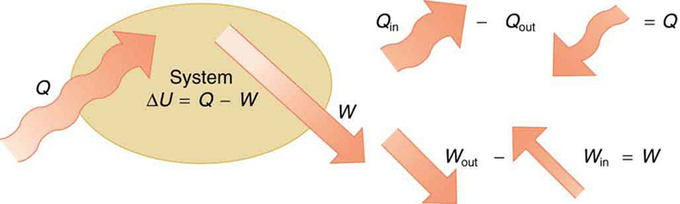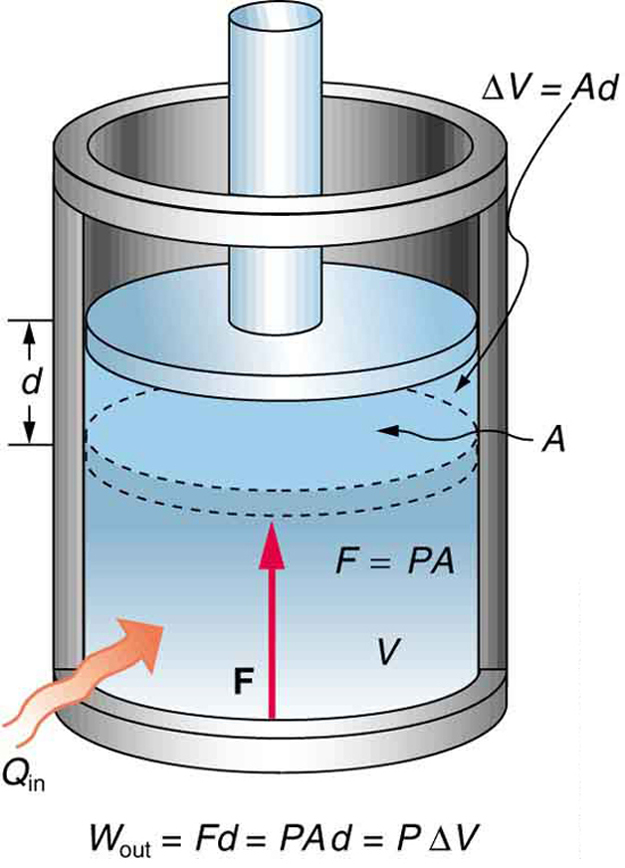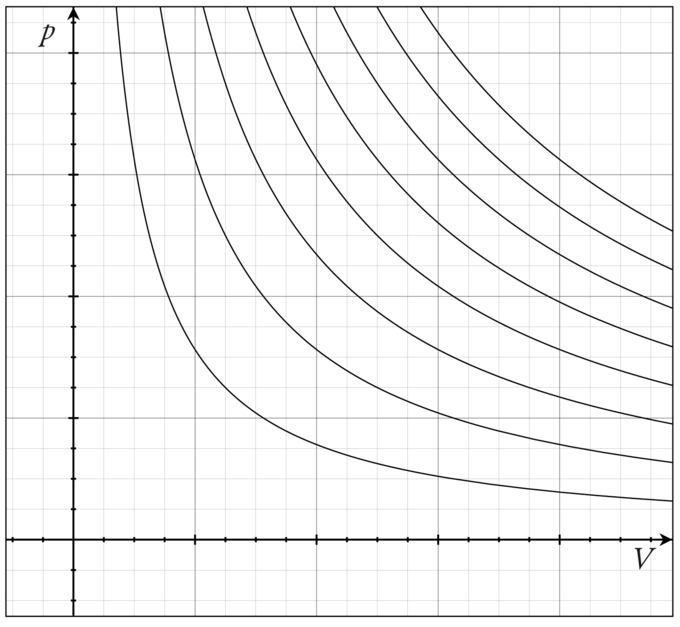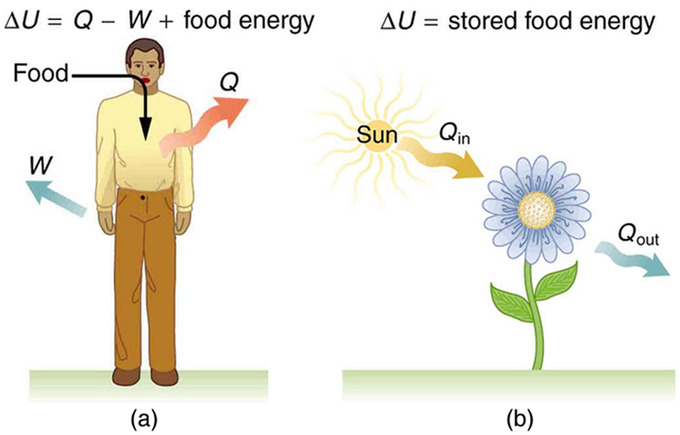## The First Law

The 1st law of thermodynamics states that internal energy change of a system equals net heat transfer minus net work done by the system.

### Learning Objectives

Explain how the net heat transferred and net work done in a system relate to the first law of thermodynamics

### Key Takeaways

#### Key Points

• The first law of thermodynamics is a version of the law of conservation of energy, specialized for thermodynamical systems.
• In equation form, the first law of thermodynamics is $\Delta \text{U} = \text{Q} - \text{W}$.
• Heat engines are a good example of the application of the 1st law; heat transfer into them takes place so that they can do work.

#### Key Terms

• internal energy: The sum of all energy present in the system, including kinetic and potential energy; equivalently, the energy needed to create a system, excluding the energy necessary to displace its surroundings.
• heat: energy transferred from one body to another by thermal interactions
• law of conservation of energy: The law stating that the total amount of energy in any isolated system remains constant, and cannot be created or destroyed, although it may change forms.

The first law of thermodynamics is a version of the law of conservation of energy specialized for thermodynamic systems. It is usually formulated by stating that the change in the internal energy of a closed system is equal to the amount of heat supplied to the system, minus the amount of work done by the system on its surroundings. The law of conservation of energy can be stated like this: The energy of an isolated system is constant.

First Law of Thermodynamics: In this video I continue with my series of tutorial videos on Thermal Physics and Thermodynamics. It’s pitched at undergraduate level and while it is mainly aimed at physics majors, it should be useful to anybody taking a first course in thermodynamics such as engineers etc..

If we are interested in how heat transfer is converted into work, then the conservation of energy principle is important. The first law of thermodynamics applies the conservation of energy principle to systems where heat transfer and doing work are the methods of transferring energy into and out of the system. In equation form, the first law of thermodynamics isInternal Energy: The first law of thermodynamics is the conservation-of-energy principle stated for a system where heat and work are the methods of transferring energy for a system in thermal equilibrium. Q represents the net heat transfer—it is the sum of all heat transfers into and out of the system. Q is positive for net heat transfer into the system. W is the total work done on and by the system. W is positive when more work is done by the system than on it. The change in the internal energy of the system, ΔU, is related to heat and work by the first law of thermodynamics, ΔU=Q−W.

$\Delta \text{U} = \text{Q} -\text{W}$.

Here ΔU is the change in internal energy U of the system, Q is the net heat transferred into the system, and W is the net work done by the system. We use the following sign conventions: if Q is positive, then there is a net heat transfer into the system; if W is positive, then there is net work done by the system. So positive Q adds energy to the system and positive W takes energy from the system. Thus ΔU=Q−W. Note also that if more heat transfer into the system occurs than work done, the difference is stored as internal energy. Heat engines are a good example of this—heat transfer into them takes place so that they can do work.

## Constant Pressure and Volume

Isobaric process is one in which a gas does work at constant pressure, while an isochoric process is one in which volume is kept constant.

### Learning Objectives

Contrast isobaric and isochoric processes

### Key Takeaways

#### Key Points

• An isobaric process occurs at constant pressure. Since the pressure is constant, the force exerted is constant and the work done is given as PΔV.
• An isobaric expansion of a gas requires heat transfer to keep the pressure constant.
• An isochoric process is one in which the volume is held constant, meaning that the work done by the system will be zero. The only change will be that a gas gains internal energy.

#### Key Terms

• internal energy: The sum of all energy present in the system, including kinetic and potential energy; equivalently, the energy needed to create a system, excluding the energy necessary to displace its surroundings.

According to the first law of thermodynamics, heat transferred to a system can be either converted to internal energy or used to do work to the environment. A process in which a gas does work on its environment at constant pressure is called an isobaric process, while one in which volume is kept constant is called an isochoric process.

### Isobaric Process (Constant Pressure)

An isobaric process occurs at constant pressure. Since the pressure is constant, the force exerted is constant and the work done is given as PΔV. An example would be to have a movable piston in a cylinder, so that the pressure inside the cylinder is always at atmospheric pressure, although it is isolated from the atmosphere. In other words, the system is dynamically connected, by a movable boundary, to a constant-pressure reservoir. If a gas is to expand at a constant pressure, heat should be transferred into the system at a certain rate. This process is called an isobaric expansion.Fig 1: An isobaric expansion of a gas requires heat transfer during the expansion to keep the pressure constant. Since pressure is constant, the work done is PΔV.

### Isochoric Process (Constant Volume)

An isochoric process is one in which the volume is held constant, meaning that the work done by the system will be zero. It follows that, for the simple system of two dimensions, any heat energy transferred to the system externally will be absorbed as internal energy. An isochoric process is also known as an isometric process or an isovolumetric process. An example would be to place a closed tin can containing only air into a fire. To a first approximation, the can will not expand, and the only change will be that the gas gains internal energy, as evidenced by its increase in temperature and pressure. Mathematically,

$\Delta \text{Q}= \Delta \text{U}$.

We may say that the system is dynamically insulated, by a rigid boundary, from the environment.

## Isothermal Processes

An isothermal process is a change of a thermodynamic system, in which the temperature remains constant.

### Learning Objectives

Identify the typical systems in which an isothermal process occurs

### Key Takeaways

#### Key Points

• For an ideal gas, the product of pressure and volume (PV) is a constant if the gas is kept at isothermal conditions.
• For an ideal gas, the work involved when a gas changes from state A to state B through an isothermal process is given as $\text{W}_{\text{A}\to \text{B}} = \text{nRT}\ln{\frac{\text{V}_\text{B}}{\text{V}_\text{A}}}$.
• For many systems, if the temperature is held constant, the internal energy of the system also is constant. It follows that Q =-W in this case.

#### Key Terms

• reversible: Capable of returning to the original state without consumption of free energy and increase of entropy.
• ideal gas: A hypothetical gas whose molecules exhibit no interaction and undergo elastic collision with each other and with the walls of the container.
• Boyle’s law: The observation that the pressure of an ideal gas is inversely proportional to its volume at constant temperature.

An isothermal process is a change of a system, in which the temperature remains constant: ΔT = 0. This typically occurs when a system is in contact with an outside thermal reservoir ( heat bath), and the change occurs slowly enough to allow the system to continually adjust to the temperature of the reservoir through heat exchange. In contrast, an adiabatic process is where a system exchanges no heat with its surroundings (Q = 0). (See our atom on “Adiabatic Process. “) In other words, in an isothermal process, the value ΔT = 0 but Q ≠ 0, while in an adiabatic process, ΔT ≠ 0 but Q = 0.

### Ideal Gas in an Isothermal Process

For an ideal, the product of pressure and volume (PV) is a constant if the gas is kept at isothermal conditions. (This is historically called Boyle’s law. ) However, the cases where the product PV is an exponential term, does not comply. The value of the constant is nRT, where n is the number of moles of gas present and R is the ideal gas constant. In other words, the ideal gas law PV = nRT applies. This means that

$\text{P} = \frac{\text{nRT}}{\text{V}} = \frac{\text{constant}}{\text{V}}$

holds.

### Calculation of Work

In thermodynamics, the work involved when a gas changes from state A to state B is simply

$\text{W}_{\text{A}\to \text{B}} = \int_{\text{V}_\text{A}}^{\text{V}_\text{B}}\text{P}\,\text{dV}$.

For an isothermal, reversible process, this integral equals the area under the relevant pressure-volume isotherm, and is indicated in blue in for an ideal gas. Again, P = nRT / V applies and with T being constant (as this is an isothermal process), we haveWork Done by Gas During Expansion: The blue area represents “work” done by the gas during expansion for this isothermal change.

$\text{W}_{\text{A}\to \text{B}} = \text{nRT}\int_{\text{V}_\text{A}}^{\text{V}_\text{B}}\frac{1}{\text{V}}\text{dV} = \text{nRT}\ln{\frac{\text{V}_\text{B}}{\text{V}_\text{A}}}$.

It is also worth noting that, for many systems, if the temperature is held constant, the internal energy of the system also is constant, and so $\Delta \text{U} = 0$. From the first law of thermodynamics, it follows that $\text{Q} =-\text{W}$ for this same isothermal process.

An adiabatic process is any process occurring without gain or loss of heat within a system.

### Learning Objectives

Assess the environments in which isothermal processes typically occur

### Key Takeaways

#### Key Points

• Adiabatic processes can occur if the container of the system has thermally-insulated walls or the process happens in an extremely short time.
• For an adiabatically expanding ideal monatomic gas which does work on its environment (W is positive), internal energy of the gas should decrease.
• In a sense, isothermal process can be considered as the opposite extreme of adiabatic process. In isothermal processes, heat exchange is slow enough so that the system’s temperature remains constant.

#### Key Terms

• Boyle’s law: The observation that the pressure of an ideal gas is inversely proportional to its volume at constant temperature.
• ideal gas: A hypothetical gas whose molecules exhibit no interaction and undergo elastic collision with each other and with the walls of the container.
• reversible: Capable of returning to the original state without consumption of free energy and increase of entropy.

An isothermal process is a change of a system, in which the temperature remains constant: ΔT = 0. This typically occurs when a system is in contact with an outside thermal reservoir (heat bath), and the change occurs slowly enough to allow the system to continually adjust to the temperature of the reservoir through heat exchange. In contrast, an adiabatic process is where a system exchanges no heat with its surroundings (Q = 0). (See our atom on “Adiabatic Process. “) In other words, in an isothermal process, the value ΔT = 0 but Q ≠ 0, while in an adiabatic process, ΔT ≠ 0 but Q = 0.

### Ideal Gas in an Isothermal Process

For an ideal, the product of pressure and volume (PV) is a constant if the gas is kept at isothermal conditions. (This is historically called Boyle’s law. ) However, the cases where the product PV is an exponential term, does not comply. The value of the constant is nRT, where n is the number of moles of gas present and R is the ideal gas constant. In other words, the ideal gas law PV = nRT applies. This means that

$\text{P} = \frac{\text{nRT}}{\text{V}} = \frac{\text{constant}}{\text{V}}$

holds. The family of curves generated by this equation is shown in. Each curve is called an isotherm.Isotherms of an Ideal Gas: Several isotherms of an ideal gas on a PV diagram.

### Calculation of Work

In thermodynamics, the work involved when a gas changes from state A to state B is simply

$\text{W}_{\text{A}\to \text{B}} = \int_{\text{V}_\text{A}}^{\text{V}_\text{B}}\text{P}\,\text{dV}$.

For an isothermal, reversible process, this integral equals the area under the relevant pressure-volume isotherm, and is indicated in blue in for an ideal gas. Again, P = nRT / V applies and with T being constant (as this is an isothermal process), we haveWork Done by Gas During Expansion: The blue area represents “work” done by the gas during expansion for this isothermal change.

$\text{W}_{\text{A}\to \text{B}} = \text{nRT}\int_{\text{V}_\text{A}}^{\text{V}_\text{B}}\frac{1}{\text{V}}\text{dV} = \text{nRT}\ln{\frac{\text{V}_\text{B}}{\text{V}_\text{A}}}$.

It is also worth noting that, for many systems, if the temperature is held constant, the internal energy of the system also is constant, and so $\Delta \text{U} = 0$. From the first law of thermodynamics, it follows that $\text{Q} =-\text{W}$ for this same isothermal process.

## Human Metabolism

The 1st law of thermodynamics explains human metabolism: the conversion of food into energy that is used by the body to perform activities.

### Learning Objectives

Contrast catabolism and anabolism in regards to energy

### Key Takeaways

#### Key Points

• Human metabolism is a complicated process. The 1st law of thermodynamics describes the beginning and ending points of these processes.
• Our body loses internal energy. There are three places this internal energy can go—to heat transfer, to doing work, and to stored fat.
• Our body provides a good example of irreversible processes. Although body fat can be converted to do work and produce heat transfer, work done on the body and heat transfer into it cannot be converted to body fat.

#### Key Terms

• metabolism: The complete set of chemical reactions that occur in living cells.
• oxidation: A reaction in which the atoms of an element lose electrons and the valence of the element increases.
• calorie: The energy needed to increase the temperature of 1 kilogram of water by 1 kelvin. It is equivalent to 1,000 (small) calories.

Metabolism in humans is the conversion of food into energy, which is then used by the body to perform activities. It is an example of the first law of thermodynamics in action. Considering the body as the system of interest, we can use the first law to examine heat transfer, doing work, and internal energy in activities ranging from sleep to heavy exercise. For example, one major factor in such activities is body temperature—normally kept constant by heat transfer to the surroundings, meaning that Q is negative (i.e., our body loses heat). Another factor is that the body usually does work on the outside world, meaning that W is positive. Thus, in such situations the body loses internal energy, since ΔU=Q−W is negative.

### Eating

Now consider the effects of eating. The body metabolizes all the food we consume. Eating increases the internal energy of the body by adding chemical potential energy. In essence, metabolism uses an oxidation process in which the chemical potential energy of food is released. This implies that food input is in the form of work. Food energy is reported in a special unit, known as the Calorie. This energy is measured by burning food in a calorimeter, which is how the units are determined.

### Catabolism and Anabolism

Catabolism is the pathway that breaks down molecules into smaller units and produces energy. Anabolism is the building up of molecules from smaller units. Anabolism uses up the energy produced by the catabolic break down of your food to create molecules more useful to your body.

### Internal energy

Our body loses internal energy, and there are three places this internal energy can go—to heat transfer, to doing work, and to stored fat (a tiny fraction also goes to cell repair and growth). As shown in Fig 1 heat transfer and doing work take internal energy out of the body, and then food puts it back. If you eat just the right amount of food, then your average internal energy remains constant. Whatever you lose to heat transfer and doing work is replaced by food, so that, in the long run, ΔU=0. If you overeat repeatedly, then ΔU is always positive, and your body stores this extra internal energy as fat. The reverse is true if you eat too little. If ΔU is negative for a few days, then the body metabolizes its own fat to maintain body temperature and do work that takes energy from the body. This process is how dieting produces weight loss.Metabolism: (a) The first law of thermodynamics applied to metabolism. Heat transferred out of the body (Q) and work done by the body (W) remove internal energy, while food intake replaces it. (Food intake may be considered as work done on the body. ) (b) Plants convert part of the radiant heat transfer in sunlight to stored chemical energy, a process called photosynthesis.

### Metabolism

Life is not always this simple, as any dieter knows. The body stores fat or metabolizes it only if energy intake changes for a period of several days. Once you have been on a major diet, the next one is less successful because your body alters the way it responds to low energy intake. Your basal metabolic rate is the rate at which food is converted into heat transfer and work done while the body is at complete rest. The body adjusts its basal metabolic rate to compensate (partially) for over-eating or under-eating. The body will decrease the metabolic rate rather than eliminate its own fat to replace lost food intake. You will become more easily chilled and feel less energetic as a result of the lower metabolic rate, and you will not lose weight as fast as before. Exercise helps with weight loss because it produces both heat transfer from your body and work, and raises your metabolic rate even when you are at rest.

### Irreversibility

The body provides us with an excellent indication that many thermodynamic processes are irreversible. An irreversible process can go in one direction but not the reverse, under a given set of conditions. For example, although body fat can be converted to do work and produce heat transfer, work done on the body and heat transfer into it cannot be converted to body fat. Otherwise, we could skip lunch by sunning ourselves or by walking down stairs. Another example of an irreversible thermodynamic process is photosynthesis. This process is the intake of one form of energy—light—by plants and its conversion to chemical potential energy. Both applications of the first law of thermodynamics are illustrated in. One great advantage of such conservation laws is that they accurately describe the beginning and ending points of complex processes (such as metabolism and photosynthesis) without regard to the complications in between.